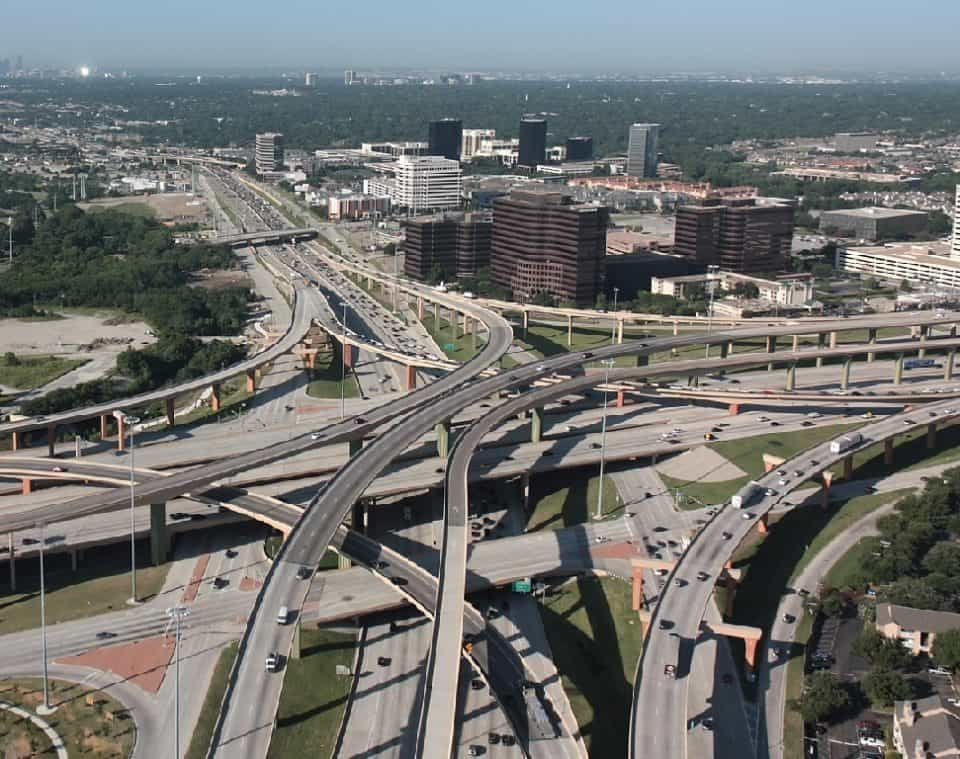Breaking News
Home / Land Surveying / Transition Curve In Highways – Purpose-Requirements-Example

# Transition Curve In Highways – Purpose-Requirements-Example

## Transition Curve

Transition curve as the name suggests, is usually a horizontal curve in plan provided to allow transition from a straight alignment to a circular curve gradually. In other words, it is a curve which connects a with infinite radius and radius R. Transition curves are usually provided between a straight and curved track/roads.Transition Curves employed in interchanges in the USA

## Objectives or Purpose

Transition curves are provided to serve following purposes. To provide Super Elevation in a gradual manner and to reduce the radius from infinity at a straight junction to a radius R at curved junction gradually. Transition curves also reduces the effect of sudden jerk on passengers due to the effect of centrifugal force induced on vehicles at curves.

### What is Difference Between Whole Circle Bearing and Quadrantal Bearing

An ideal Transition curve should satisfy following requirements,

• The curve should be tangential at its junction points so that the radius of transition is infinity at straight junction and R at the curved junction.
• Rate of change of super-elevation is equal to the rate of change of curvature so that full super-elevation can be provided within the length of transition curve.

Spirals and clothoids are mostly adopted to provide transition curves, as suggested by design codes. Apart from these, cubic parabolic curves and lemniscates are also used as per alignment requirements. Governing parameters for setting out these curves are the acceleration of vehicles, the required super elevations, lengths available for transition and deflection angles. Empirical formulas are used to design transition curves.Transition Curve – Straight to Curve Transformation

### Example Transition Curve Calculation

Determine the length of transition curve for a BG track having 4deg curvature and super-elevation of 12 cm. The maximum permissible speed on the curve is 85kmph.

Radius = 1720/ 4 deg = 430m

maximum speed allowed on track, Martin’s formula

Vmax1 = 4.35x sqrt(430- 67) = 82.87kmph

Max Cant deficiency for BG  = 7.6cm

Theoretical super-elevation= SE act + D = 12+ 7.6 = 19.6 cm = 0.196m

Vmax2 = sqrt (127xRxSE th/G) = sqrt(127 x 430 x 0.196/ 1.676) = 79.9

therefore maximum speed allowed  Vmax = min(82.87,85,79.9)  = 79kmph

### Calculation Of Super Elevation

Length of Transition curve

L =  4.4 sqrt(R)= 4.4 sqrt(430) = 91.2m

L = 3.6 SE = 3.6*12 = 43.2m

L (as per rate of change of radial acc.) = 3.28 * V^3 / R = 3.28 *  (0.278 * 79)^3 / 430 = 80.80m

Therefore Length of TC ~92m

.

## Land Surveying & Architects

### THANKS.